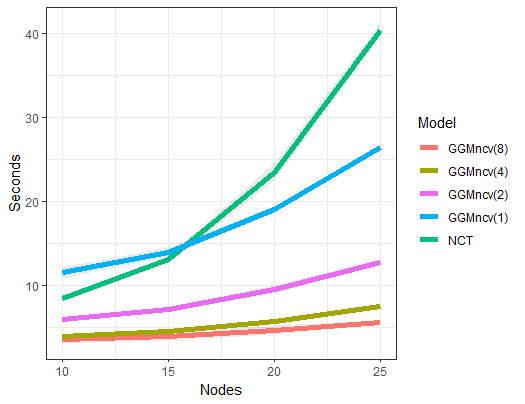# Introduction

It is important to note that nct is based on the NCT function in the R package NetworkComparisonTest. Key extensions to the methodology are nonconvex regularization, the de-sparsified glasso estimator, additional default tests (e.g., KL-divergence), and user-defined test-statistics, to name but a few.

Furthermore, nct should be much faster for two primary reasons:

1. glassoFast, introduced in (Sustik and Calderhead 2012), is used instead of glasso.

2. Parallel computing via parallel’s parLapply, whereas NetworkComparisonTest uses a serially executed for loop (with cores = 1, GGMncv uses lapply)

The gains in speed will be most notable in larger networks, as shown in the following comparison.

# CPU Time

In the following, time, as a function of network size, is investigated for several cores compared to NCT. Note that all settings in GGMncv are set to be comparable to NCT, for example, using lasso and the number of tuning parameters (i.e., 50). One important distinction is that GGMncv compute 4 default test-statistic instead of 2 (as in NCT).

library(GGMncv)
library(dplyr)
library(ggplot2)
library(NetworkComparisonTest)

p <- seq(10, 25, 5)

sim_fun <- function(x){

main <- gen_net(p = x, edge_prob = 0.1)

y1 <- MASS::mvrnorm(n = 500,
mu = rep(0, x),
Sigma = main$cors) y2 <- MASS::mvrnorm(n = 500, mu = rep(0, x), Sigma = main$cors)

st_1 <- system.time({
fit_1 <- nct(Y_g1 = y1,
Y_g2 = y2,
iter = 1000,
desparsify = FALSE,
penalty = "lasso",
cores = 1,
progress = FALSE)
})

st_2 <- system.time({
fit_1 <- nct(Y_g1 = y1,
Y_g2 = y2,
iter = 1000,
desparsify = FALSE,
penalty = "lasso",
cores = 2,
progress = FALSE)
})

st_4 <- system.time({
fit_1 <- nct(Y_g1 = y1,
Y_g2 = y2,
iter = 1000,
desparsify = FALSE,
penalty = "lasso",
cores = 4,
progress = FALSE)
})

st_8 <- system.time({
fit_1 <- nct(Y_g1 = y1,
Y_g2 = y2,
iter = 1000,
desparsify = FALSE,
penalty = "lasso",
cores = 8,
progress = FALSE)
})

st_NCT <- system.time({

fit_NCT <- NCT(data1 = y1,
data2 = y2,
it = 1000,
progressbar = FALSE)
})

ret <- data.frame(
times =  c(st_1, st_2,
st_4, st_8, st_NCT),
models = c("one", "two",
"four", "eight", "NCT"),
nodes = x
)

return(ret)

}

sim_res <- list()

reps <- 5

for(i in seq_along(p)){
print(paste("nodes", p[i]))

sim_res[[i]] <- do.call(rbind.data.frame,
replicate(reps, sim_fun(p[i]),
simplify = FALSE))
}

do.call(rbind.data.frame, sim_res) %>%
group_by(models, nodes) %>%
summarise(mu = mean(times),
std = sd(times)) %>%
ggplot(aes(x = nodes, y = mu, group = models)) +
theme_bw() +
geom_ribbon(aes(ymax = mu + std, ymin = mu - std),
alpha = 0.1) +
geom_line(aes(color = models), size = 2) +
ylab("Seconds") +
xlab("Nodes") +
scale_color_discrete(name = "Model",
labels = c("GGMncv(8)",
"GGMncv(4)",
"GGMncv(2)",
"GGMncv(1)",
"NCT"),
breaks = c("eight",
"four",
"two",
"one",
"NCT"))The performance gains are clear, especially when using 8 cores with GGMncv.